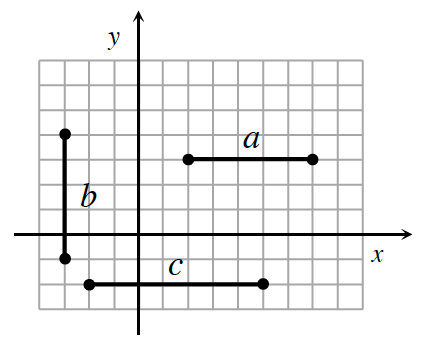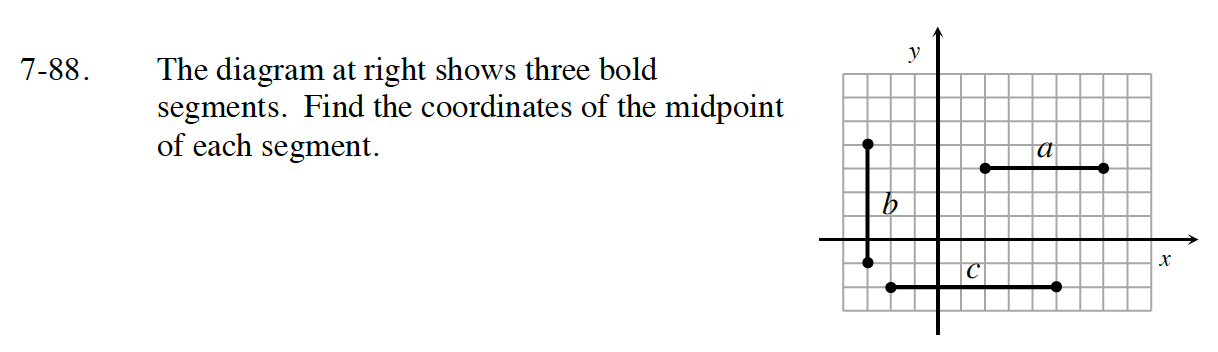Home > CC4 > Chapter 7 > Lesson 7.2.1 > Problem7-88

7-88.
1. The diagram below shows three bold segments. Find the coordinates of the midpoint of each segment. Homework Help ✎Start at the endpoints of each segment and move to the center for each line. What are the coordinates of this point.
When calculating midpoint, find the average of the x-coordinates and the average of the y-coordinates.